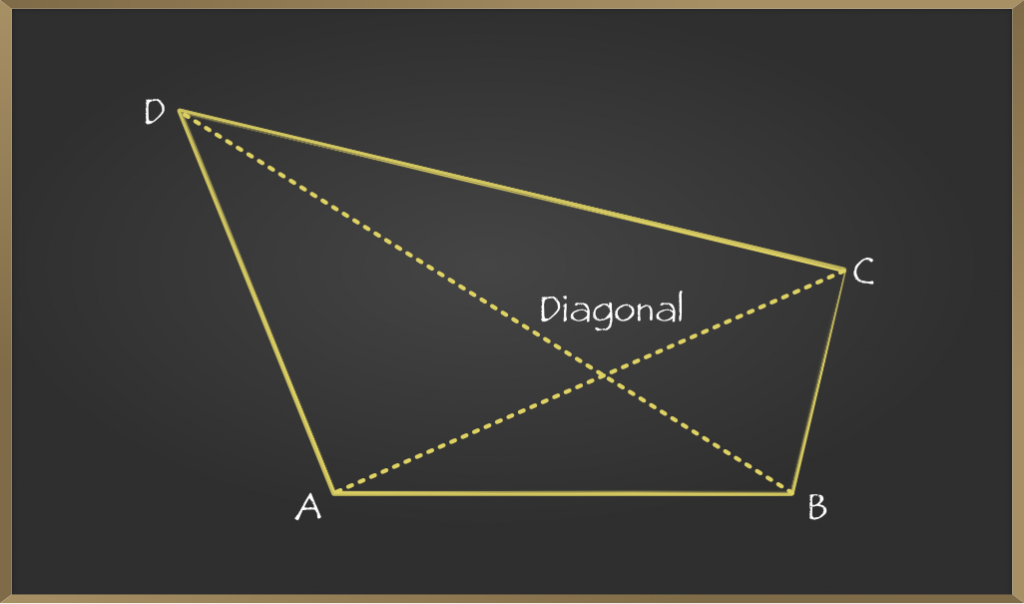# Types of Quadrilaterals and their PropertiesThere are basically two properties of quadrilaterals – a quadrilateral should be closed shape with four sides and all internal angles should sum up to 360. But how do we distinguish one

quadrilateral shape with another? There are five types of quadrilaterals based on the shape – rectangle, square, rhombus, parallelogram and trapezium. Let’s discuss each of these five quadrilaterals. In detail, a rectangle is a quadrilateral with four right angles thus all the angles in a rectangle are equal that is 90 degree. Moreover the opposite sides of the rectangle are parallel and equal and diagonals bisect each other. Some real-world examples of rectangles are dollar bills, license, bars and many more. Moving on, a rectangle has three properties: all the angles of a rectangle are 90 degree, opposite sides of a rectangle are equal and balanced, and lastly diagonals of the rectangle bisect each other. If we want to calculate the area and the perimeter of the rectangle then the area of the rectangle equals length into breadth and perimeter of rectangle equals length plus breadth. The second type of quadrilateral is a square.

It has four equal sides and angles and is also a regular quadrilateral as both its sides and angles are equal just like a rectangle. A square has four angles of 90 degree each thus it can also be a rectangle whose two adjacent sides are equal. Some of the real-life examples are a chessboard, dice, the waffles, the Rubik’s Cube, carom board and many more. Moving on to the properties of square, a square has three properties: all the angles of the square are 90-degree, all sides of the square are equal and parallel to each other and the diagonals bisect each other perpendicularly. If we want to calculate the area and perimeter of the square with side a then area of square equals to a into a equals to a square: a x a = a^2

and perimeter of the square equals to = 2 a + a) = 4a. The next type of quadrilateral is a rhombus.   It is a quadrilateral whose all four sides are equal in length and opposite sides are parallel to each

Other. However the angles are not equal to 90 degrees, because rhombus with the right angles would become a square. Another name for rhombus is diamond as it looks like a diamond in playing Cards. Let’s move onto the properties of rhombus : it has four properties – opposite angles are equal, both sides are equal and opposite sides are parallel to each other,  diagonals bisect each other perpendicularly and the sum of any two edges and angle is 180 degree. If we want to calculate the area and perimeter of rhombus with side a and two diagonals d1 and d2 then the perimeter of rhombus equals 4a.

## Area of rhombus equals 1/2 x D1 x D2

The next type of quadrilateral is a parallelogram. As the name suggests, it is a simple quadrilateral whose opposite sides are parallel thus it has two pairs of parallel sides. Moreover the opposite angles in a parallelogram are equal and its diagonals bisect each other. The doclet office building in Hamburg Germany is one of the examples of a parallelogram. In fact a rectangle, square and rhombuses are all parallelograms. thus any object that has one of these shapes is a parallelogram. Lets move on to the properties of a parallelogram. It has four properties: opposite angles are equal, opposite sides are equal and parallel diagonals bisect each other and the sum of any two edges at an angle is 180 degrees.

If we want to calculate the area and perimeter of a parallelogram then the perimeter of a parallelogram equals two x length + breadth and area of a parallelogram equals length into height. The last quadrilateral that we will talk about is a trapezium. It has only one pair of parallel sides referred to as bases and the other two sides are called legs or lateral sides. For example, take pyramids. That’s a triangle but imagine if you take out the top part of it we get the trapezium. Similarly, have you noticed that when you go out for a movie night and cannot stop munching on that popcorn? Most of those popcorn bags are in the shape of the trapezium. Having said that, let’s talk about the properties and area formula of the trapezium.  A trapezium is a quadrilateral where only one pair of opposite sides are parallel to each other. To calculate the area and parameter of trapezium where H is the height of a trapezium, then the parameter of trapezium equals sum of length of all the sides and the area of the trapezium equals half into sum of lengths of parallel sides into H.